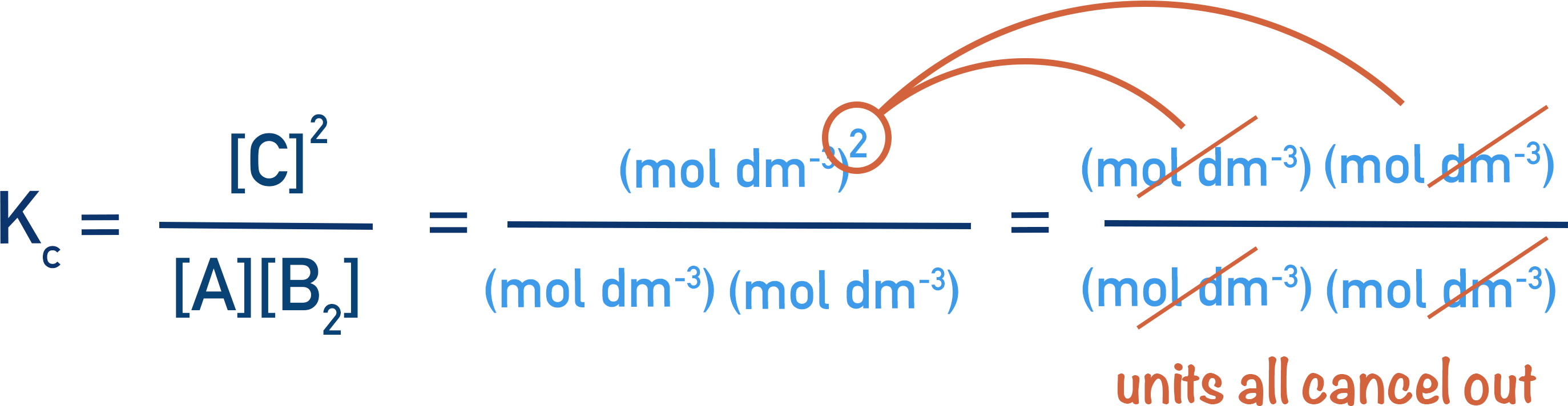Kc Calculations

By using the concentrations of reactants and products at equilibrium, Kc can be determined.

For example:1.0 moles of each A and B2 are allowed to reach equilibrium at temperature, T. 0.5 moles of C are present at equilibrium, determine Kc.

Write out the Kc expression.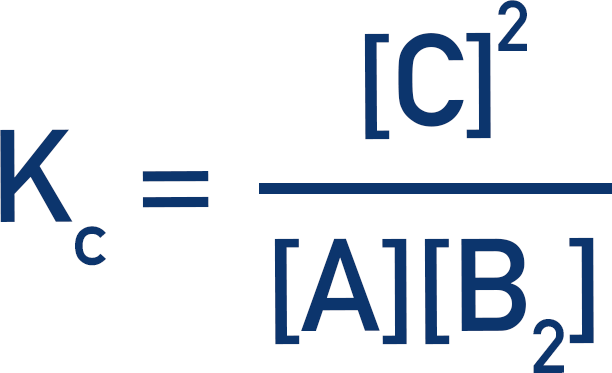If we know the starting amounts of A and B2, and the final amount of C, we can find the amounts at equilibrium.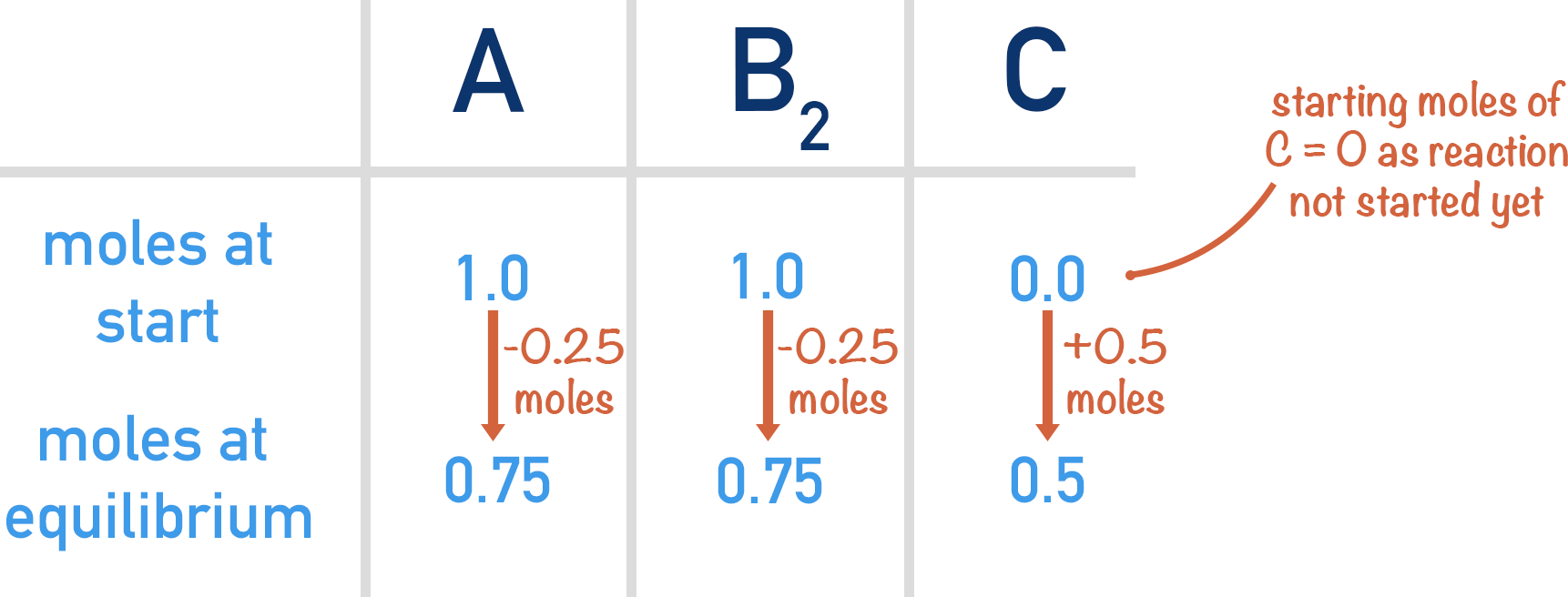Two moles of C are produced for every one mole of each A and B2 that react. Therefore, if 0.5 moles of C are present at equilibrium, 0.25 moles of each A and B2 must have reacted.One mole of each A and B2 are present at the start. If 0.25 moles of each react together, 0.75 moles of each are left over. This means that at equilibrium we have 0.75 moles of each A and B2, and 0.5 moles of C.

These values can now be used in the Kc expression to find the value of Kc.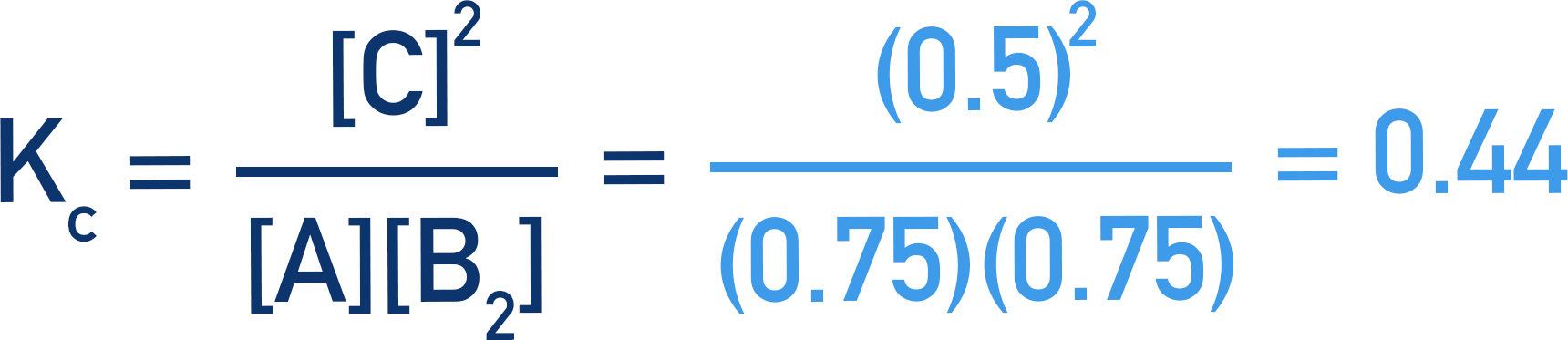Here, the number of moles of A, B2 and C are used instead of concentrations. This is because the volume of the system does not change, so the volumes cancel out.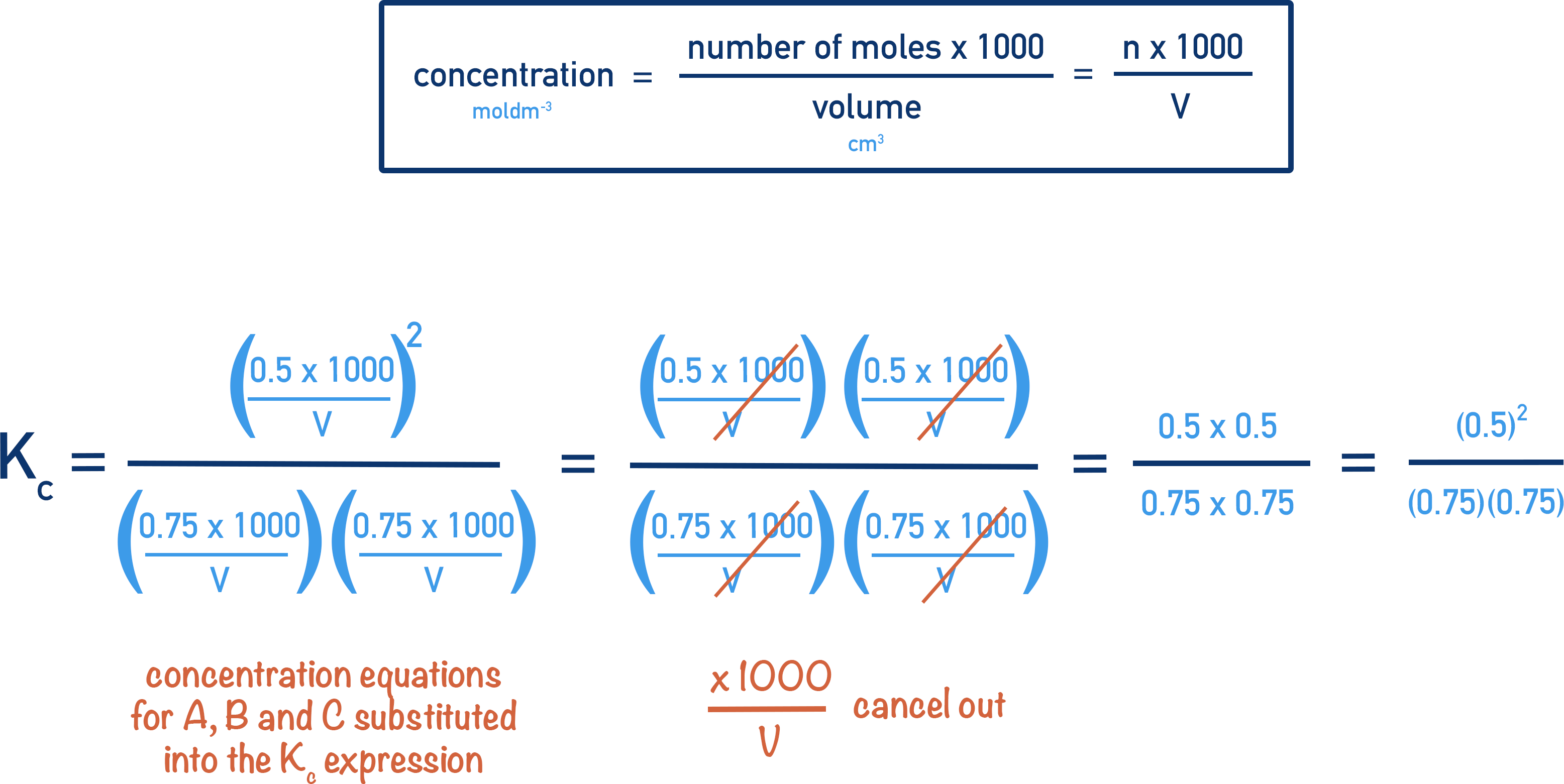There are no units for Kc in this example because the units for concentration also cancel out.